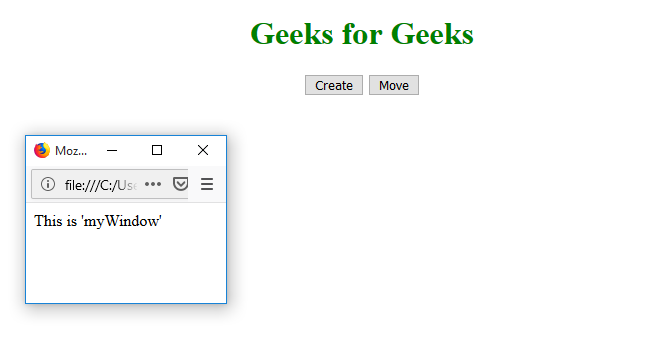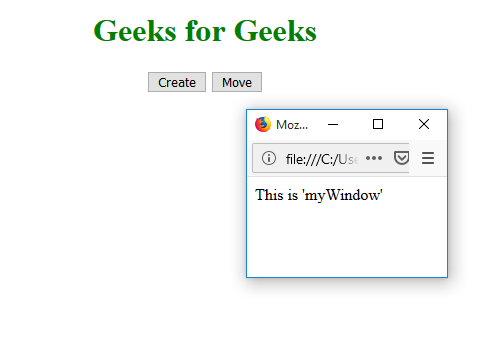Related Articles
HTML | Window moveTo() Method
• Difficulty Level : Basic
• Last Updated : 26 Jul, 2019

The moveTo() method is used in the window to moves the window from the left and top coordinates.

Syntax:

`window.moveTo(x, y)`

Parameter:

• x: A positive or negative number that specifies the horizontal coordinate to be moved to
• y: A positive or negative number specifies the vertical coordinate to be moved to

Example: Move the window.

 ` ` `<``html``> ` ` `  `<``head``> ` `    ``<``title``> ` `      ``Window moveTo() Method ` `    `` ` `    ``<``style``> ` `        ``h1 { ` `            ``color: green; ` `        ``} ` `    `` ` ` ` ` `  `<``body``> ` `    ``<``center``> ` `        ``<``h1``>Geeks for Geeks ` `        ``<``button` `onclick``=``"openWin()"``> ` `          ``Create ` `      `` ` `        ``<``button` `onclick``=``"moveWin()"``> ` `          ``Move ` `      `` ` ` `  `        ``<``script``> ` `            ``var myWindow; ` ` `  `            ``function openWin() { ` `                ``myWindow =  ` `                  ``window.open("", ` `                              ``"myWindow", ` `                              ``"width=200", ` `                              ``"height=100"); ` `               `  `                ``myWindow.document.write( ` `                  ``"<``p``>This is 'myWindow'"); ` `            ``} ` ` `  `            ``function moveWin() { ` `                ``myWindow.moveTo(500, 100); ` `                ``myWindow.focus(); ` `            ``} ` `        `` ` `    `` ` ` ` ` `  ` `

Output:
Initial:New window:Move window:Supported Browsers: The browser supported by Window moveTo() Method are listed below:

• Goole Chrome
• Mozilla Firefox
• Opera
• Safari
• EdgeMy Personal Notes arrow_drop_up
Recommended Articles
Page :